### Day 20 - Product/Quotient Rule - 09.14.15

Update

Bell Ringer
1. Find the derivative of the following: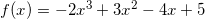1.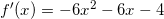2.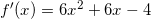3.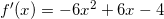4.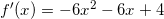5. none of the above

2. Find the second derivative (derivative of the derivative) of the following:1.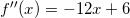2.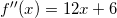3.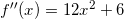4.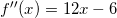5. none of the above

3. Find the point(s) at which the following function has a horizontal tangent line: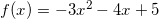1.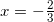2.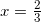3.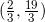4.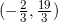5. none of the above

4. Find the derivative of the following function using the Product Rule: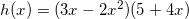1.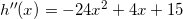2.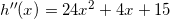3.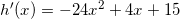4.5. none of the above

5. Find the derivative of the following function: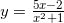1.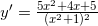2.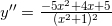3.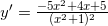4.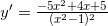5. none of the above

Review
• Pre-calculus
• Slope
• Equation of a Line
• Secant Line vs. Tangent Line (video)
• Tangent Line
• How can the slope of one point be found?
• Finding the derivative of polynomials using limits (example)
• Basic Differentiation Rules
• How can derivatives be calculated using basic differentiation rules?
• Product Rule/Quotient Rule
• How can derivatives of the product/quotient of functions be calculated

Lesson

Exit Ticket
• Posted on the board at the end of the block.

Homework
• WNQ the following:

Lesson Objectives
• How can the Product Rule and Quotient Rule be used to find derivatives?

#### In-Class Help Requests

Standard(s)
• APC.5
• Investigate derivatives presented in graphic, numerical, and analytic contexts and the relationship between continuity and differentiability.
• The derivative will be defined as the limit of the difference quotient and interpreted as an instantaneous rate of change.
• APC.6
• ​The student will investigate the derivative at a point on a curve.
• Includes:
• finding the slope of a curve at a point, including points at which the tangent is vertical and points at which there are no tangents
• using local linear approximation to find the slope of a tangent line to a curve at the point
• ​defining instantaneous rate of change as the limit of average rate of change
• approximating rate of change from graphs and tables of values.